FanDuel Championship Odds
+600
6 to 1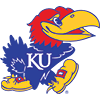Kansas
8.7% implied probability

+900
9 to 1Gonzaga
6.1% implied probability

+1100
11 to 1Baylor
5.1% implied probability

+1300
13 to 1Dayton
4.4% implied probability

+1500
15 to 1Michigan State
3.8% implied probability

+1600
16 to 1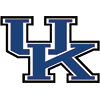Kentucky
3.6% implied probability

+1700
17 to 1Duke
3.4% implied probability

+1700
17 to 1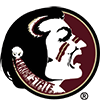Florida State
3.4% implied probability

+2000
20 to 1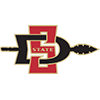San Diego State
2.9% implied probability

+2000
20 to 1Maryland
2.9% implied probability

+2200
22 to 1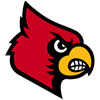Louisville
2.7% implied probability

+2200
22 to 1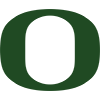Oregon
2.7% implied probability

+2500
25 to 1Villanova
2.4% implied probability

+2800
28 to 1Creighton
2.1% implied probability

+2800
28 to 1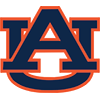Auburn
2.1% implied probability

+3200
32 to 1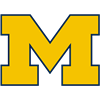Michigan
1.9% implied probability

+3200
32 to 1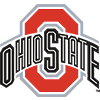Ohio State
1.9% implied probability

+4500
45 to 1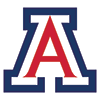Arizona
1.3% implied probability

+4500
45 to 1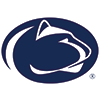Penn State
1.3% implied probability

+4500
45 to 1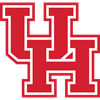Houston
1.3% implied probability

+4500
45 to 1West Virginia
1.3% implied probability

+5000
50 to 1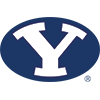BYU
1.2% implied probability

+5500
55 to 1Illinois
1.1% implied probability

+5500
55 to 1Virginia
1.1% implied probability

+5500
55 to 1Butler
1.1% implied probability

+6000
60 to 1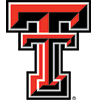Texas Tech
1.0% implied probability

+6500
65 to 1Iowa
0.9% implied probability

+7500
75 to 1St. Mary's (CAL)
0.8% implied probability

+7500
75 to 1Utah State
0.8% implied probability

+7500
75 to 1Xavier
0.8% implied probability

+7500
75 to 1Wichita State
0.8% implied probability

+7500
75 to 1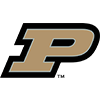Purdue
0.8% implied probability

+7500
75 to 1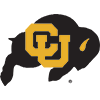Colorado
0.8% implied probability

+7500
75 to 1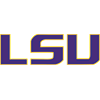Louisiana State
0.8% implied probability

+8000
80 to 1Providence
0.8% implied probability

+8500
85 to 1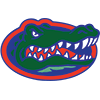Florida
0.7% implied probability

+8500
85 to 1Memphis
0.7% implied probability

+8500
85 to 1Wisconsin
0.7% implied probability

+8500
85 to 1Marquette
0.7% implied probability

+9000
90 to 1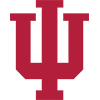Indiana
0.7% implied probability

+10000
100 to 1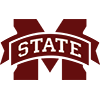Mississippi State
0.6% implied probability

+10000
100 to 1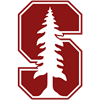Stanford
0.6% implied probability

+10000
100 to 1VCU
0.6% implied probability

+10000
100 to 1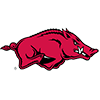Arkansas
0.6% implied probability

+10000
100 to 1Connecticut
0.6% implied probability

+10000
100 to 1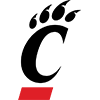Cincinnati
0.6% implied probability

+11000
110 to 1North Carolina State
0.6% implied probability

+11000
110 to 1Rhode Island
0.6% implied probability

+11000
110 to 1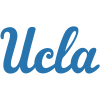UCLA
0.6% implied probability

+12000
120 to 1Yale
0.5% implied probability

+12000
120 to 1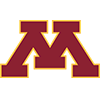Minnesota
0.5% implied probability

+12000
120 to 1Northern Iowa
0.5% implied probability

No changes have been recorded yet.
+12000
120 to 1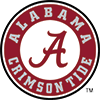Alabama
0.5% implied probability

+12000
120 to 1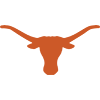Texas
0.5% implied probability

+12000
120 to 1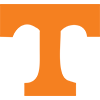Tennessee
0.5% implied probability

+15000
150 to 1E. Tennessee State
0.4% implied probability

+15000
150 to 1Liberty
0.4% implied probability

+15000
150 to 1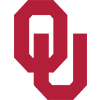Oklahoma
0.4% implied probability

+15000
150 to 1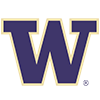Washington
0.4% implied probability

+15000
150 to 1Notre Dame
0.4% implied probability

+18000
180 to 1Georgetown
0.3% implied probability

+18000
180 to 1Clemson
0.3% implied probability

+18000
180 to 1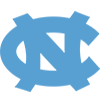North Carolina
0.3% implied probability

+23000
230 to 1USC
0.3% implied probability

+23000
230 to 1Syracuse
0.3% implied probability

+23000
230 to 1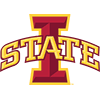Iowa State
0.3% implied probability

+23000
230 to 1Virginia Tech
0.3% implied probability

+25000
250 to 1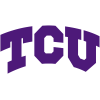TCU
0.2% implied probability

+25000
250 to 1New Mexico State
0.2% implied probability

+25000
250 to 1Pittsburgh
0.2% implied probability

+25000
250 to 1Oregon State
0.2% implied probability

+25000
250 to 1Saint Louis
0.2% implied probability

No changes have been recorded yet.
+25000
250 to 1SMU
0.2% implied probability

No changes have been recorded yet.
+25000
250 to 1Wright State
0.2% implied probability

No changes have been recorded yet.
+25000
250 to 1Wofford
0.2% implied probability

No changes have been recorded yet.
+25000
250 to 1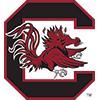South Carolina
0.2% implied probability

No changes have been recorded yet.
+25000
250 to 1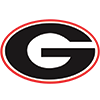Georgia
0.2% implied probability

+25000
250 to 1Buffalo
0.2% implied probability

+25000
250 to 1Washington State
0.2% implied probability

No changes have been recorded yet.
+25000
250 to 1Arizona State
0.2% implied probability

+25000
250 to 1Belmont
0.2% implied probability

No changes have been recorded yet.
+25000
250 to 1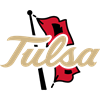Tulsa
0.2% implied probability

No changes have been recorded yet.
+25000
250 to 1Nevada
0.2% implied probability

No changes have been recorded yet.
+25000
250 to 1St. John's
0.2% implied probability

+25000
250 to 1Temple
0.2% implied probability

+25000
250 to 1Vermont
0.2% implied probability

+25000
250 to 1Davidson
0.2% implied probability

+25000
250 to 1Stephen F. Austin
0.2% implied probability

No changes have been recorded yet.
+25000
250 to 1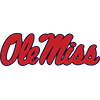Mississippi
0.2% implied probability

+25000
250 to 1Utah
0.2% implied probability

No changes have been recorded yet.
+25000
250 to 1Missouri
0.2% implied probability

+25000
250 to 1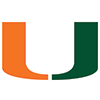Miami
0.2% implied probability